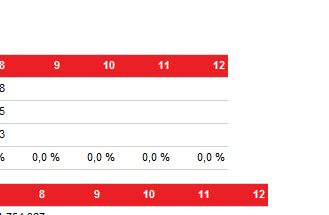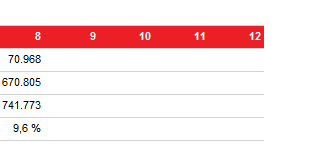Home / Reporting Services / Prevent Divide by Zero in SSRSPrevent Divide by Zero in SSRS

In a previous post, I wrote about how to prevent the divide by zero issues in Reporting Services. The problem with the custom code I used was that the code returned the value 0 when one of the numbers is 0 or unknown.

So when the outcoming was NULL, the code returned 0. This result in the value 0 in my reports:I have found a better code on the internet to prevent the divide by zero error in SSRS.

Old code:

Public Function Divide(ByVal first As Double, ByVal second As Double) As Double
If second = 0 OR first = 0
Return 0
Else
Return first / second
End If
End Function

Usage in an expression: Code.Divide(Value A, Value B)

Better Code:
Public Function CalcDivision(ByVal Numerator As Object, _
ByVal Denominator As object, ByVal DivideByZeroDefault As Object) As Object
If Denominator <> 0 Then
Return Numerator/Denominator
Else
Return DivideByZeroDefault
End If
End Function

Usage in an expression: =Code.CalcDivision(Field A, Field B,Nothing)

So it return NULL and not 0! Better solution!I hope Microsoft will make it easier to fix these kind of thing with less effort. I haven’t tried it in SQL 2014/2016 yet so maybe the already have a solution to prevent Divide By Zero. They should include some cool function by default in SSRS or something like that!

How to Refresh Reporting Services Cache?

I am working for a client which uses a Datawarehouse in which the data is …

One comment

1.=IIf(Isnothing(Sum(Fields!Expr2.Value))=true OR Sum(Fields!Expr2.Value)=0 ,”NULL”,Sum(Fields!Expr1.Value)/Sum(Fields!Expr2.Value))